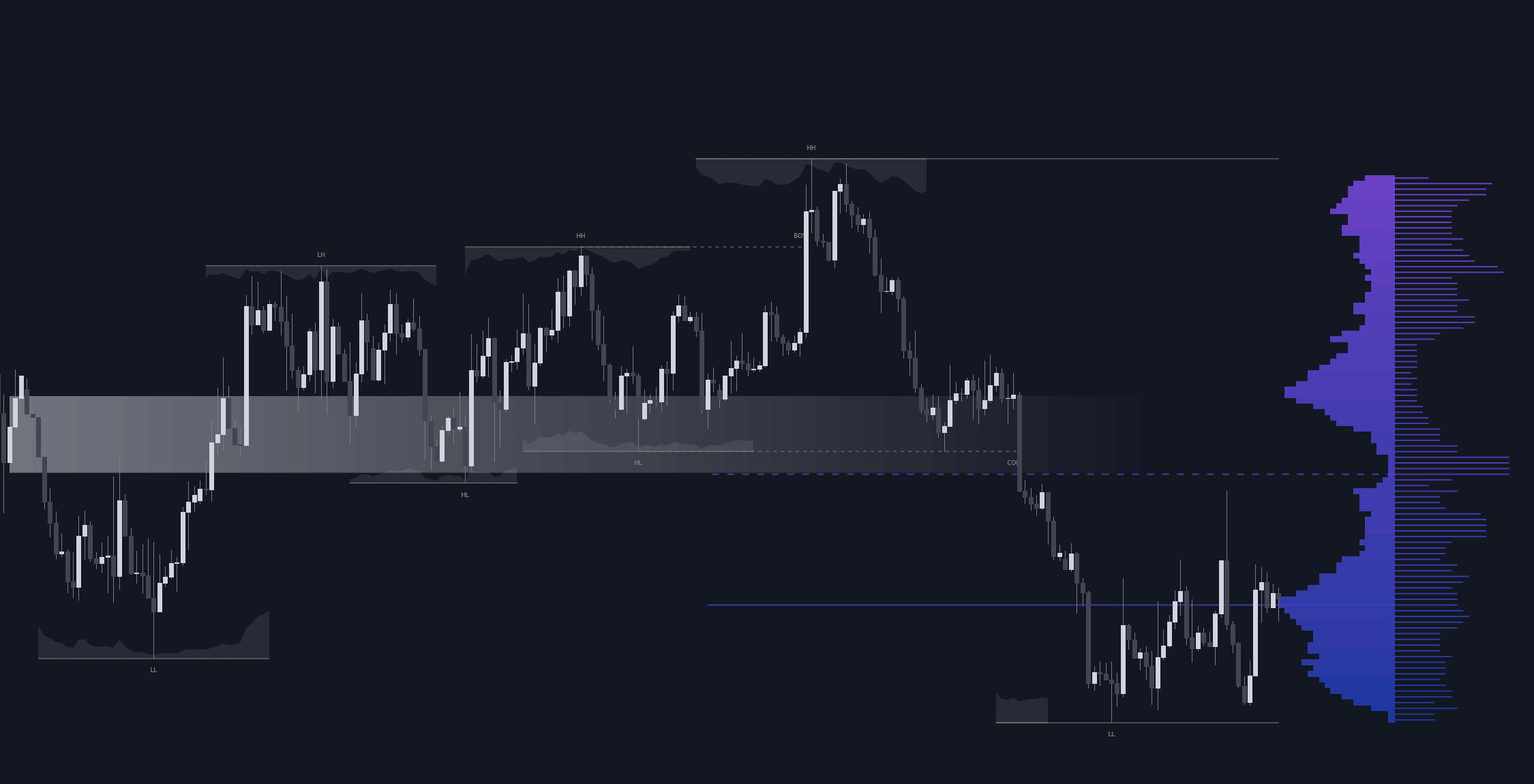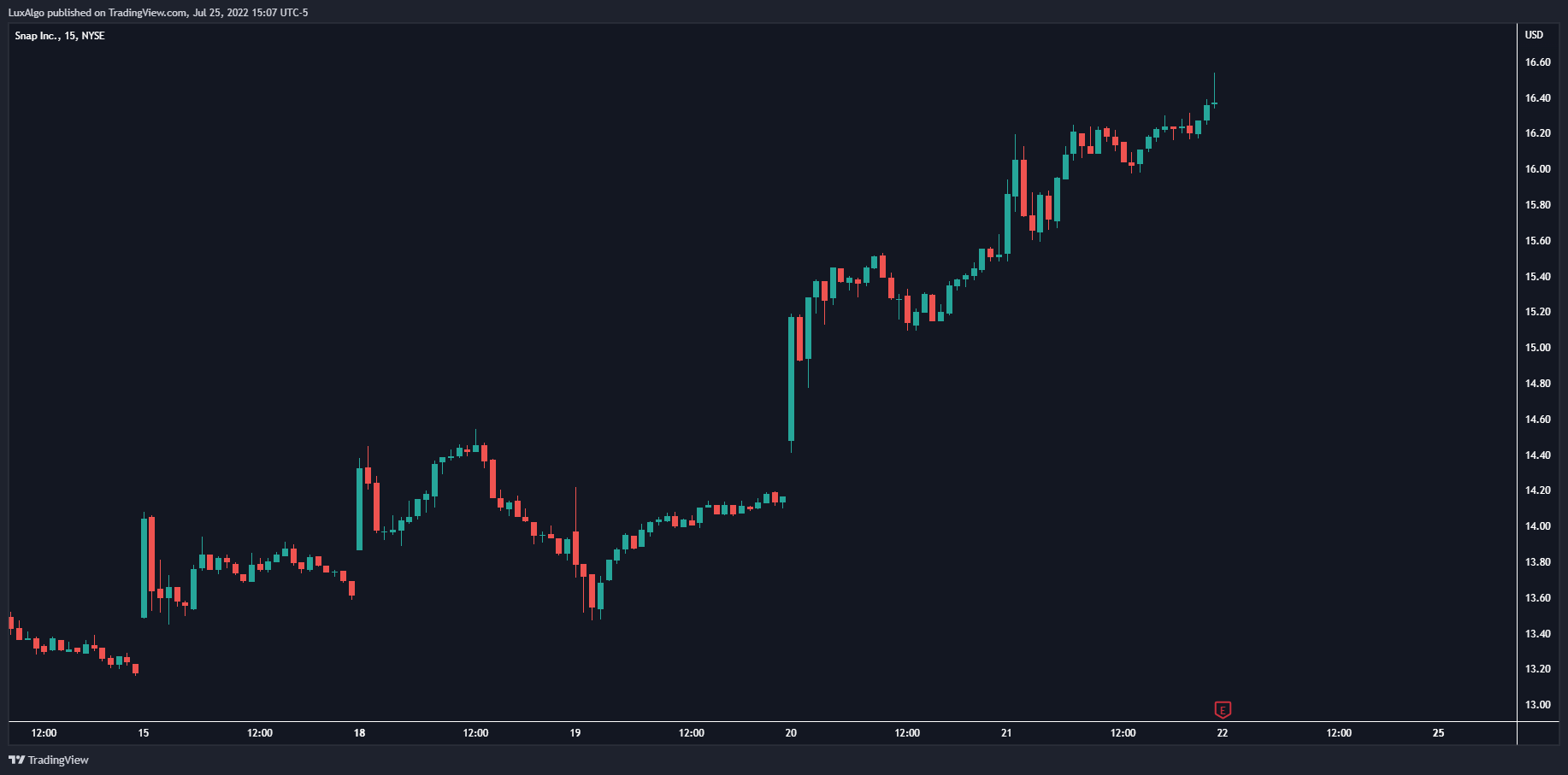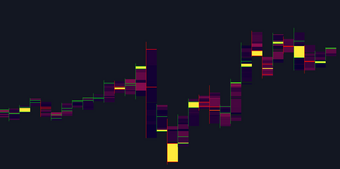# A Deep Dive Into Moving Averages

Moving averages are inherent in the world of technical analysis and are present in the core calculations of many technical indicators. In this post, we take a deep dive into 3 types of moving averages used every day by traders: the Simple Moving Average ( SMA ), Exponential Moving Average ( EMA ) and the Weighted Moving Average ( WMA ).

Moving averages are inherent in the world of technical analysis and are present in the core calculations of many technical indicators. In this post, we take a deep dive into 3 types of moving averages used every day by traders: the Simple Moving Average ( SMA ), Exponential Moving Average ( EMA ) and the Weighted Moving Average ( WMA ).

The topics covered below can have practical applications while others are solely informative.

#### Introduction

Moving averages are trend indicators commonly used to smooth the closing prices by removing or attenuating certain variations and are able to estimate underlying trends. Their usage can be recorded as early as 1829 by John Finlaison for smoothing mortality rates (1).

In technical analysis moving averages are often essential for traders and can be found in every technical analysis software. However, they are not specific to this field as they often appear in Time Series Analysis and Digital Signal Processing (DSP).

Moving averages possess a single user setting that generally determines the degree of smoothness. This setting is often referred to as the moving average "length", "period" or less commonly "window size".

#### Curiosities About The Simple Moving Average

The Simple Moving Average abbreviated to "SMA", also known as the "Arithmetic Moving Average" or "Moving/Rolling Mean/Average" is certainly the most well-known moving average due to its simplicity and numerous applications in other domains. The SMA with period length is commonly calculated as follows:

Here all the weights w would be equal to 1/length (which is why we often state that a SMA has uniform weights).

##### Relationship With The Momentum Oscillator

Changes in a simple moving average with period length are equal to a momentum oscillator of the same period divided by length, that is:

This can be explained from the calculations of the changes in a Simple Moving Average:

The closing prices with the same lag cancel each other out, leaving only C(t) and C(t-length) divided by length in the final calculation.

As such you could tell whether a moving average of period length is rising or declining by simply comparing the current closing price to the closing price length bars ago. If the current closing price is higher; then the moving average is rising, else it is declining.

This relationship allows us to efficiently compute the SMA , allowing us to obtain a computation time independent of the moving average period which is very important for real-time high-frequency applications of the SMA .

##### Lag Of The SMA

Lag is defined as the effect moving averages have to return past price variations instead of new ones. For most moving averages this amount of lag can be quantified as the weighted sum between the moving average weights w(i) and the time lag associated with them. Higher weights given to more recent values would return a moving average with less lag.

All the weights of a simple moving average are equal to 1/length. The lag of a Simple Moving Average is thus given by:

As such, the lag (in bars) of a Simple Moving Average is equal to its period minus 1, divided by 2.

Offsetting an SMA Lag bars in the past allows us to have it centered with the price.

Using an SMA as input for another SMA would return a smoother output; this process is known as cascading. In the case of the Simple Moving Average , cascading many SMAs of the same period would converge toward a Gaussian function.

The Irwin–Hall Probability Density Function can describe the result of cascading multiple SMAs using an impulse as input.

#### Curiosities About The Exponential Moving Average

The Exponential Moving Average ; abbreviated as "EMA", also known as an "Exponentially Weighted Moving Average" or "Exponential Average" is a recursive moving average. That is, it uses a previous output for its computation.

This moving average is slightly more reactive than the Simple Moving Average due to its lower degree of filtering.

An EMA of period length is calculated as follows:

or

with smoothing constant a = 2/(length+1).

##### Traders Prefer The EMA Over The SMA

The trading community seems to have developed a preference for the EMA over the SMA . This might be explained by the superior reactivity of the EMA over the SMA .

The EMA is also more commonly used in the creation of technical indicators, sometimes for its superior reactivity, its computational efficiency, or sometimes simply by preference.

Several studies attempted to indicate which moving average (between the EMA and SMA ) provided better performances. The conclusion can vary depending on the markets and methodology used. Dzikevičius & Šaranda found superior results of the EMA over the SMA (2), while Predipbhai found better results from an EMA-based MACD over an SMA-based one (3).

##### The EMA Helps Avoiding Division By Zero

In scenarios where we are required to perform a division with a moving average applied to a denominator, the EMA can help to avoid division by 0 as long as the smoothing factor is lower than 1 ( EMA period superior to 1)

For a< 1, the EMA has an exponentially decaying infinite impulse response. The impulse response converges towards 0 but never reaches it.

This can be useful if we want to obtain the ratio between the average upward variations and average downward variations. In the event where there is a significant number of upward variations, an SMA of the downward variations might eventually be equal to 0; the EMA prevents this.

##### The EMA Has The Same Lag As An SMA

We previously mentioned that the EMA is more reactive than the SMA , but quantifying the lag of an EMA from the weighted sum between the EMA weights and their associated lag gives the same results as the lag of an SMA .

The weights of an EMA can be obtained from its impulse response, which is described as:

The lag is then calculated as follows:

#### Curiosities About The Weighted Moving Average

The Weighted Moving Average ; abbreviated as "WMA", also known as a Linearly Weighted Moving Average (LWMA), is the most reactive moving average when compared to the SMA and EMA . The WMA uses linearly decaying weights for its calculations, giving higher weights to more recent prices.

The WMA can be calculated as follows:

##### Relationship With The SMA

It's interesting to observe how certain moving averages are related to each other. In the case of the WMA and SMA , the change of a WMA of period length can be given by the difference between the price and an SMA offset by 1 bar, divided by (length+1)/2.

This equality is described as follows:

This also shows that the changes in a WMA with a period length-1 can indicate if the price is above or below an SMA of period length.

Like with the SMA , this relationship allows the calculation of the WMA efficiently allowing us to obtain a computation time independent of the moving average period.

##### Relationship With The Linear Regression

It can seem extremely surprising, but it is indeed possible to compute a simple Linear Regression of the price using linear combinations between a WMA and an SMA (under certain conditions).

The first point of a simple Linear Regression with coordinates (X1,Y1) fitted through the most recent length price observations can be obtained as follows:

While the last point with coordinates (X2,Y2) is given by:

The periods of the WMA and SMA are both equal to length. Drawing a line using the above coordinates would return the simple Linear Regression fitted to the most recent length price observations. The slope of the linear regression is equal to:

#### References

(1) Hoem, J. M. (1984). A contribution to the statistical theory of linear graduation. Insurance: Mathematics and Economics, 3(1), 1-17.

(2) Dzikevičius, A., & Šaranda, S. (2010). EMA Versus SMA usage to forecast stock markets: the case of S&P 500 and OMX Baltic Benchmark. Business: Theory and Practice, 11(3), 248-255.

(3) Predipbhai, N. P. (2013). Comparison between exponential moving average based MACD with simple moving average based MACD of technical analysis . International Journal of Scientific Research, 2(12), 189-197.

## Recent posts### Smart Money Concepts (SMC) Explained for Trading Forex, Stocks, & Crypto

##### September 9, 2022### The Best Time Frame for Trading (Actual Truth)

##### August 27, 2022### Liquidity Heatmap LTF

##### May 31, 2022